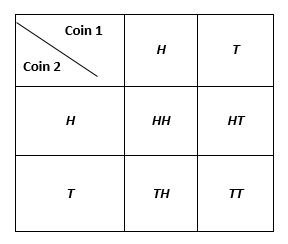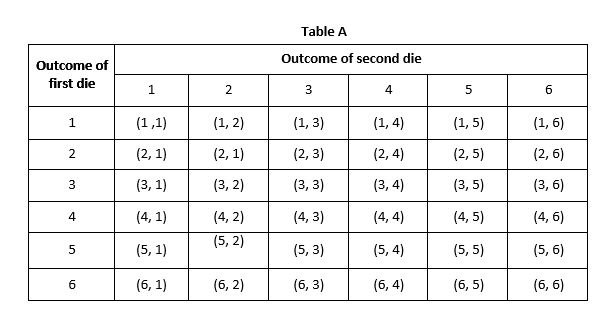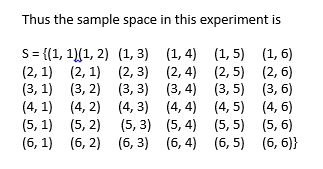Select Page

# Introduction

The idea of probability is familiar to everyone. In our daily life we make statements such as the following;

• “It is likely to rain today.”
• “I will probably go abroad this year.”
• “I am almost sure that I will win this game.”
• “The probability that nuclear war will began is very low.”

In all these statements there is lack of certainty. The theory of probability is an interesting branch of Mathematics. It has its origin in the game of chances. It plays an important role in making decisions in situations where there is lack of certainty. During the seventeenth century, the gambles call upon the mathematicians to provide them optimum strategies for various games of chances so as to increase their chances of winning. The modern theory of probability has wide applications in the fields of insurance, business and biological and physical sciences.

In the theory of probability, we introduce a common measure of expression by measuring probability between zero and one. If an event has never happened or is impossible to occur, it is assigned a probability of 0. If an event is sure to occur, it is assigned a probability of 1. For events which may or may not happen, the probability ranges between 0 and 1.

We shall start our study of the theory of probability by introducing some concepts such as experiments. Sample space, events etc.

## Random Experiment

In Statistics, the term experiment is used in a much wider sense as compared to some subjects of Sciences such as Physics and Chemistry. For the purpose of theory of probability we use this term to describe any process which generates raw data. For example, the tossing of a coin is considered an experiment or sometimes a random experiment. Other examples of experiments are; rolling of a die, selecting a bolt from a lot and observing whether it is defective or not, and many more. All such experiments have two properties in common, which are as follows:

1. Each experiment has several possible outcomes which can be specified in advance.

For example, in tossing a coin, the possible outcomes are: head and tail. In rolling a die, the possible outcomes are: 1, 2, 3, 4, 5 and 6. In selecting a bolt the possible outcomes are: defective or non-defective.

2. We are certain about the outcome or the result of the experiment.

For example, in tossing a coin, although the possible outcomes are head and tail, but we are not certain whether it will be head or a tail. Likewise, in selecting a bolt, we are not certain whether the bolt will be defective or non-defective.

## Sample Space

The set or collection of all possible outcomes of an experiment is called sample space. It is denoted by S. Each element of a sample space is called a sample point.

For example;

1. When we toss a coin, the sample space is and each of the elements H (head) and T (tail) is a sample point.
2. Similarly, when we roll a die, the sample space is and each element 1, 2, 3, 4, 5, 6 (spots on the die) is sample point.
3. In drawing a card from a pack of 52 cards, there are 52 possible outcomes. Therefore, the sample of this experiment consists of 52 sample points.
4. Suppose a coin is tossed twice or two distinct coins are tossed simultaneously. There will be 2 x 2 = 4 possible outcomes as shown in the following table. Thus the sample space is S= {HH,HT,TH,TT}.5. Suppose a coin is coin is tossed three times or three distinct coins are tossed simultaneously. There will be 2 x 2 x 2 = 8 possible outcomes. Thus the sample space of this experiment will be S={HHH,HHT,THH,HTT,THT,TTH,TTT}

6. If a die is rolled twice or two distinct dice are rolled simultaneously, there will be 6 x 6 = 36 possible outcome as shown in the following Table A.## Event

The possible outcome of an experiment is called an event. Thus an event is a subset of a sample space S. events are normally denoted by first few capital letters A, B, C etc. For example, when a coin is tossed, the sample space is S = {H, T} and the subset A = {H} is the event that a head occurs. When two coins are tossed, the sample space is S= {HH, HT, TH, TT} and the subset B = {HH, HT, TH} is the event that at least one head appears when two coins are tossed. We say that an event A occurs when any one of the outcomes in A occurs.

Since, events are sets; hence we have algebra of events corresponding to the algebra of sets considered before. By using set operations on events in sample space S we can obtain other events in S as defined below;

### 1. The Certain or Sure Events

An event comprises of the sample space S itself is the sure or certain event since an element of sample space must occur.

### 2. The Impossible Event

An event consisting of the null set  is called impossible event because a null set contains no outcome.

### 3. Mutually Exclusive Events

If the sets corresponding to events A and B are disjoints, i.e. A  B = , then the events A and B are said to be mutually exclusive. In other words, two events are mutually exclusive if they cannot both occur at the same time.

### 4. Complementary Events

The set of the possible outcomes in the sample space S which are not in the event A is the complement of A relative to S and is denoted by A’.

### 5. Union of Events

The union of two events A and B, denoted by A  B, comprises of those outcome which belongs to either A or B or both A and B.

## 6. Intersection of Events

The intersection of two events A and B, denoted by A  B, consists of those outcomes which are common to both A and B.

### 7. Difference of Events

The difference of two events A and B is the events consisting of those outcomes included in the event A but not included in the event B.

### 8. Equal events

When every outcome contained in the event A is also contained in another event B and conversely every outcome of B is also contained in A, the events are said to be equal.

### 9. Simple and Compound Events

If an event consists of only one sample point, it is called simple or elementary event. On the other hand, if the event consists more than sample points than it is called compound event. For example, when two coins are tossed, the event A = {HH} that two heads will appear is simple event but B = {HH, HT, TH} that atleast one head appears is compound event. Similarly, when two dices are rolled, the event A = {1,1} that an ace appears on both dices is a simple event, whereas, B = {(1,6), (2,5), (3,4), (4,3), (5,2), (6,1)} that the sum of two faces is 7 is compound event.

## Equally Likely, Mutually Exclusive and Exhaustive Outcomes

When each outcome of a sample space is likely to occur as any other, the outcomes are said to be equally likely. For example, if we toss a fair coin, head is likely to occur as tail. There are, therefore, equally likely outcomes. Similarly, if we roll a fair die, each of the six faces is as likely to occur as other. Hence all the six faces of a fair die are equally likely outcomes.

If occurrence of an outcome prevents the occurrence of the other outcome, i.e. if one outcome occurs, other cannot occur; they are called mutually exclusive outcomes. Suppose we toss a coin. If head occurs, the tail cannot occur. Thus, they mutually exclusive. Similarly, if we roll a die and face 4 occurs, the other faces cannot come up. Thus all the six faces of a fair die are mutually exclusive.

Outcomes are said to be exhaustive if they constitute the entire sample space. For example, if we tos a coin, the possible outcomes are head and tail. There is no other possibility; the coin will not stand on the edge. Similarly, in rolling a cubical die, there are six exhaustive outcomes which are 1, 2, 3, 4, 5 and 6.

Up Next : Probability Definition , Formula, Examples

Or

Conditional Probability and Examples

Shares